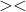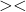## x/2-y/3=3,4x-3y=22​

Question

x/2-y/3=3,4x-3y=22in progress 0
5 months 2021-08-14T05:05:51+00:00 1 Answers 2 views 0

## Answers ( )

x = 10

y = 6

Step-by-step explanation:

x/2 – y/3 = 3 – – – (1)

4x – 3y = 22 – – – (2)

From (1):

x/2 – y/3 = 3

(3x – 2y)/6 = 3

3x – 2y = 18 – – – – (11)

4x – 3y = 22 – – – – (2)

Multiply (11) by 1.5

4.5x – 3y = 27 _____(111)

4x – 3y = 22 – – – (2)

Subtract :

0.5x = 5

x = 5 / 0.5 = 10

From :

4x – 3y = 22

Put x = 10

4(10) – 3y = 22

40 – 3y = 22

-3y = 22 – 40

-3y = – 18

y = 6